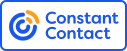# Your grades please for Southampton v Spurs - (09/03/19)

Text Block:
Please give your honest and fair assessment of the performance of all the players who took part in this game, including playing substitutes. I would prefer that those who complete this function have watched 90 minutes of the game in question.Don't forget to grade those players mentioned in the second section below.
1Here are the first ten team members:-

1 = 1 , 2 = 2 , 3 = 3 , 4 = 4 , 5 = 5 , 6 = 6 , 7 = 7 , 8 = 8 , 9 = 9 , 10 = 10
Answer
 1 2 3 4 5 6 7 8 9 10
Number of
Responses
Rating
Score*
Lloris 97 5.9
Walker-Peters 97 4.9
Sanchez 97 5.2
Vertonghen 97 5.6
Rose 97 4.3
Sissoko 97 5.5
Dier 96 5.2
Lucas Moura 96 5.5
Dele 97 6.1
Eriksen 97 5.0
*The Rating Score is the weighted average calculated by dividing the sum of all weighted ratings by the number of total responses.Show Details
2Don't forget to grade these players too!

1 = 1 , 2 = 2 , 3 = 3 , 4 = 4 , 5 = 5 , 6 = 6 , 7 = 7 , 8 = 8 , 9 = 9 , 10 = 10
Answer
 1 2 3 4 5 6 7 8 9 10
Number of
Responses
Rating
Score*
Kane 96 6.2
Son 96 5.0
Davies 94 5.1
Llorente 92 4.8
*The Rating Score is the weighted average calculated by dividing the sum of all weighted ratings by the number of total responses.Show Details
3I would also like you to give marks out of 10 in the following skill areas:-

1 = 1 , 2 = 2 , 3 = 3 , 4 = 4 , 5 = 5 , 6 = 6 , 7 = 7 , 8 = 8 , 9 = 9 , 10 = 10
Answer
 1 2 3 4 5 6 7 8 9 10
Number of
Responses
Rating
Score*
SKILL 89 5.4
PLUCK (EFFORT) 88 4.5
UNFORCED ERRORS (The less errors, the better the mark) 89 4.0
RESOLVE (Defensive ability) 88 3.6
SCORE RATIO (Goals scored in relation to shots) 89 3.1
*The Rating Score is the weighted average calculated by dividing the sum of all weighted ratings by the number of total responses.Show Details
4
Now please grade Mauricio Pochettino on three areas - Team Selection, Tactics, and Substitutions

1 = 1 , 2 = 2 , 3 = 3 , 4 = 4 , 5 = 5 , 6 = 6 , 7 = 7 , 8 = 8 , 9 = 9 , 10 = 10
Answer
 1 2 3 4 5 6 7 8 9 10
Number of
Responses
Rating
Score*
Team Selection 94 4.9
Tactics 93 4.4
Substitutions 91 4.3
*The Rating Score is the weighted average calculated by dividing the sum of all weighted ratings by the number of total responses.Show Details
5Finally, here's a chance for you to give a single mark out of ten for the performance of the officials led by Kevin Friend.

1 = 1 , 2 = 2 , 3 = 3 , 4 = 4 , 5 = 5 , 6 = 6 , 7 = 7 , 8 = 8 , 9 = 9 , 10 = 10
 1 2 3 4 5 6 7 8 9 10
Number of
Responses
Rating
Score*
91 5.8
*The Rating Score is the weighted average calculated by dividing the sum of all weighted ratings by the number of total responses.Show Details
 Online Surveys  by